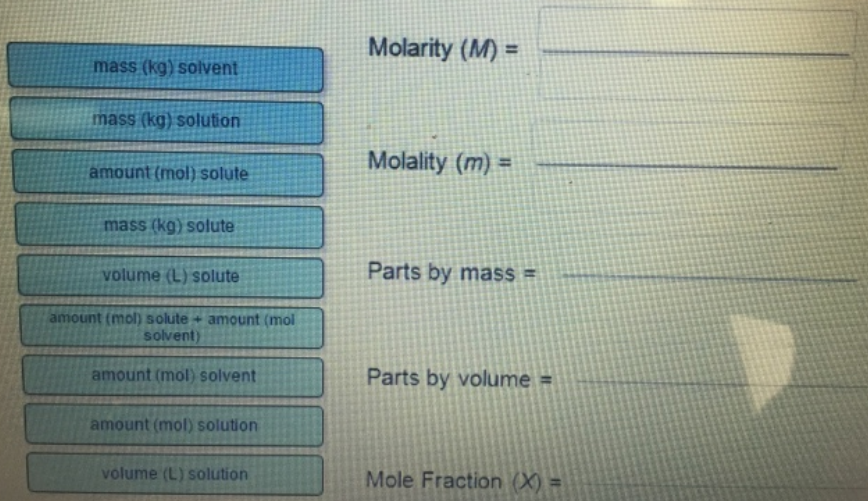# Problem: Complete each of the following concentration terms with the correct ratio of quantities.

###### FREE Expert Solution

• Molarity = mol/L
• Molality = mol/kg solvent
• parts by mass = mass solute/mass solution
• parts by volume = volume solute/volume solution
• mole fraction = mol solute/mole solution###### Problem Details

Complete each of the following concentration terms with the correct ratio of quantities.